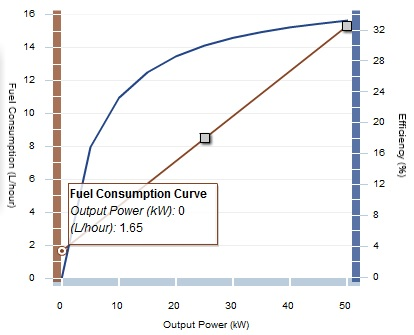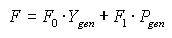# HOMER Pro 3.10

 Navigation: Glossary Generator Fuel Curve SlopeType: Input Variable Units: fuel units/hr/kW Symbol: F1

The generator fuel curve slope is the marginal fuel consumption of the generator, in units of fuel per hour per kW of output, or equivalently, units of fuel per kWh. If you plot a straight line of fuel consumption versus the power output of the generator, the slope of that line is the fuel curve slope.

For example, if a 50-kW generator consumes 8.48 L/hr at 25 kW output, and 15.3 L/hr at rated output, the slope of the fuel curve is (15.3-8.48)/(50-25) = 0.273 L/hr/kWoutput. This fuel curve is plotted below.The Fuel Curve Calculator helps calculate the fuel curve slope and intercept coefficient.

If the generator is running in a particular time step, HOMER calculates the fuel consumption rate for that time step using the following equation:where: F = fuel consumption rate this time step [L/hr] F0 rated] F1 = generator fuel curve slope [L/hr/kWoutput] Ygen = rated capacity of the generator [kW] Pgen = output of the generator in this time step [kW]

If the generator is not running in a particular time step, then the fuel consumption for that time step is zero.